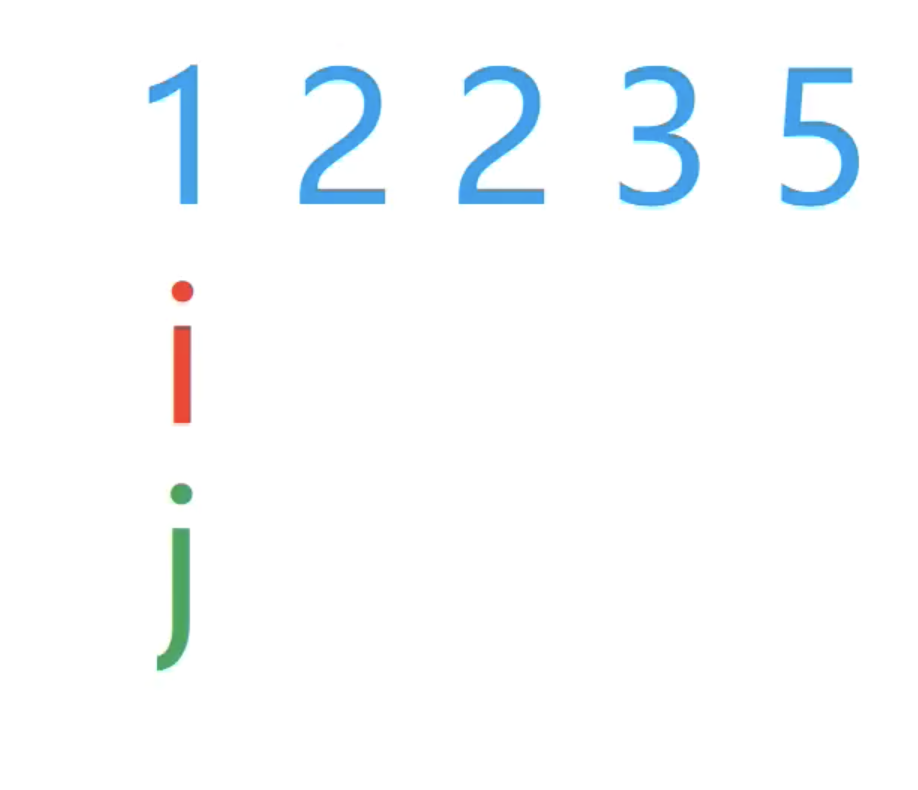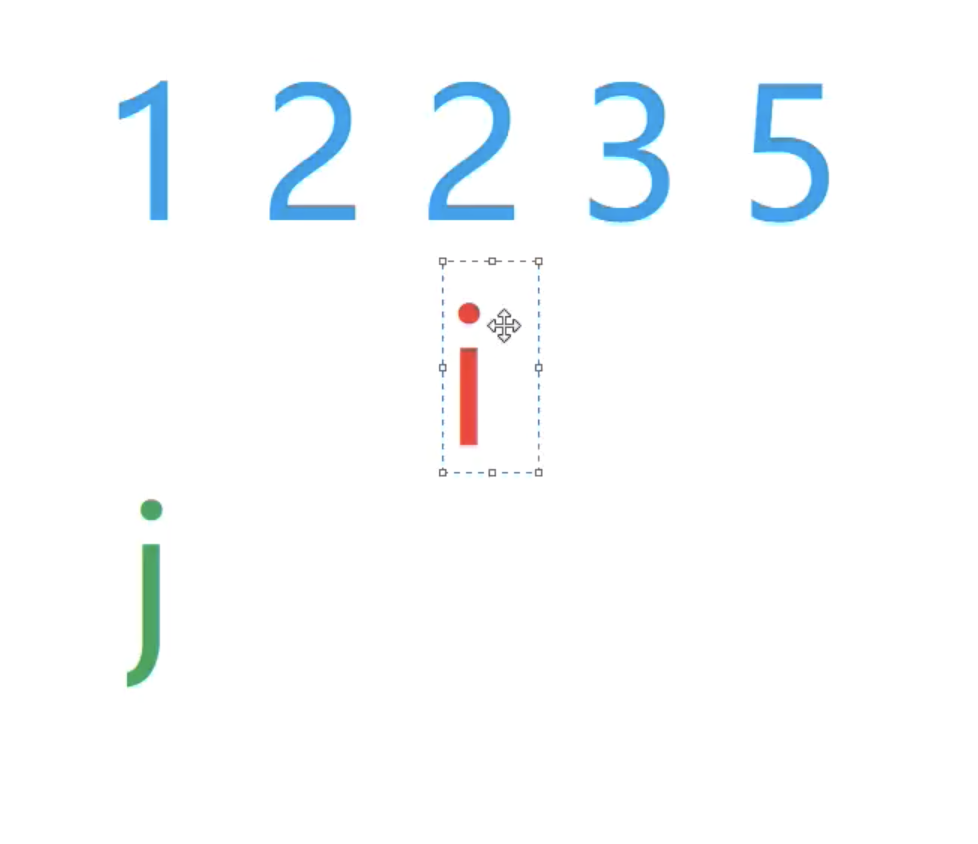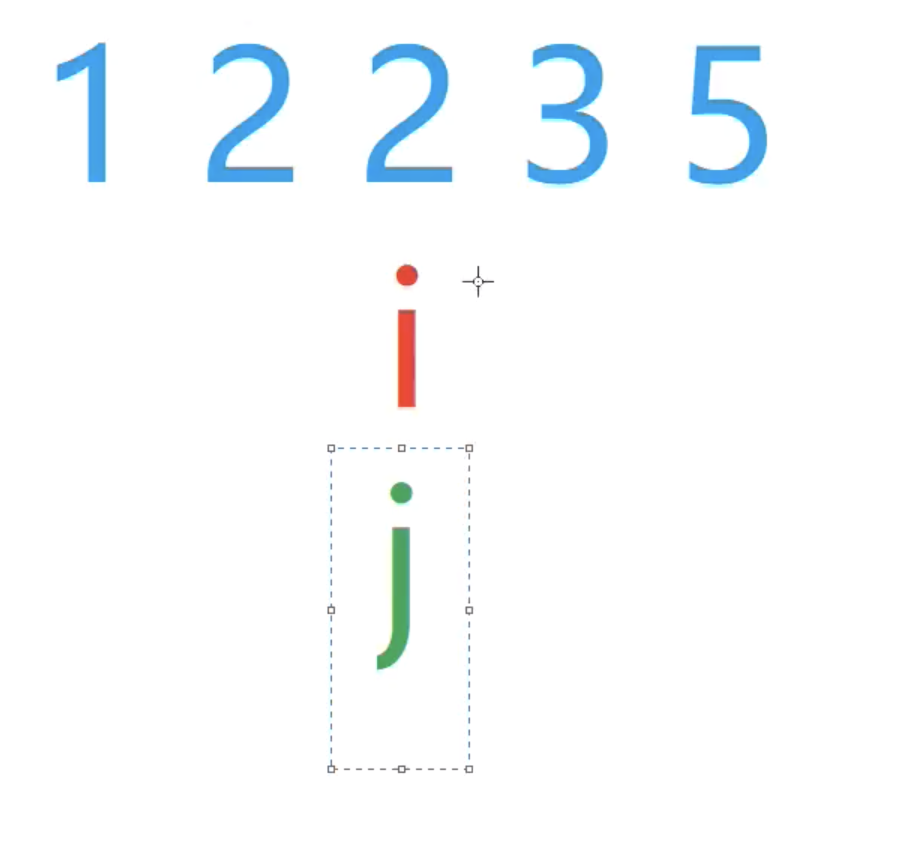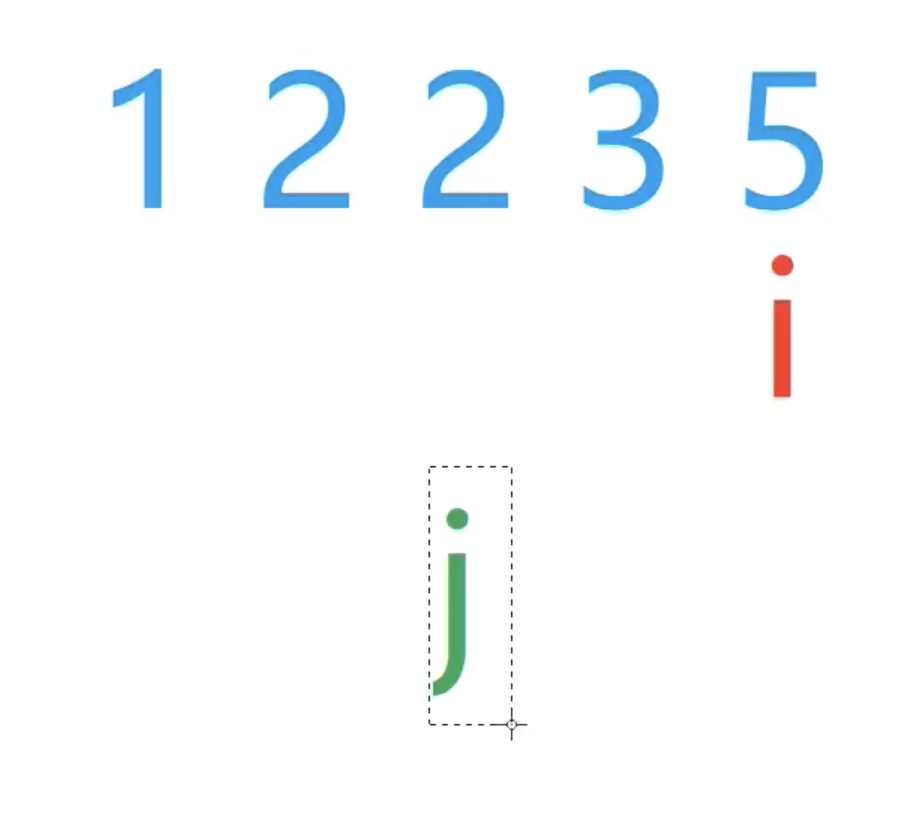• 原题链接： AcWing 799. 最长连续不重复子序列 关键词： 双指针模板题目 给定一个长度为 n 的整数序列，请找出最长的包含重复的数的连续区间，输出它的长度。
原题链接： AcWing 799. 最长连续不重复子序列
关键词： 双指针模板题
给定一个长度为 n 的整数序列，请找出最长的不包含重复的数的连续区间，输出它的长度。
输入格式
第一行包含整数 n。
第二行包含 n 个整数（均在 0∼105 范围内），表示整数序列。
输出格式
共一行，包含一个整数，表示最长的不包含重复的数的连续区间的长度。
数据范围
1≤n≤105
输入样例：
5
1 2 2 3 5

输出样例：
3


思路：
双指针算法的核心思路就是，找到某种逻辑，使得原本需要用两重循环的O(n2)的算法变为O(n)的算法。
用两个指针i、j来遍历，其中：

i从0~n-1
j从0开始， 表示为从i开始往左走能达到的不出现重复元素的最左端的位置

如何判断区间内有无重复元素？
开一个数组记录数字出现的次数，当然也可以用哈希表
做法：

用a[N]来存输入的数，用s[N]来存数出现的次数，例如s[a[i]]就表示a[i]出现的次数
外层循环i从0~n-1， 当a[j]出现的次数大于1时，说明出现了重复元素，此时内层循环将j移动到使得[i, j]不出现重复元素的最左的位置
ans取所有区间长度的最大值

时间复杂度分析：
对于i来说，移动n次；
对于j来说，每个i都有个对应的j，j变现为往右移动或者不动（j是不会往左移动的，这很好证明），最多移动n次
总共最多移动2n次，时间复杂度为O(n)

代码实现：
#include <iostream>
#include <algorithm>

using namespace std;

const int maxn = 100010;
int n;
int a[maxn], s[maxn];   //a用来存输入的数 s用来存a中数出现的个数

int main(){
int ans = 0;

cin >> n;
for(int i = 0; i < n; i ++ ) cin >> a[i];

for(int i = 0, j = 0; i < n; i ++ ){
s[a[i]]++;	//i指针右移，a[i]出现的次数+1
while(s[a[i]] > 1){ //a[i]出现的次数大于1，说明出现了重复元素
s[a[j]]--;	//a[j]出现的次数-1
j++;	//j指针右移
}
ans = max(ans, i - j + 1);
}
cout << ans << endl;

return 0;
}



展开全文• 最长连续不重复子序列的问题 应用： 1.求最长连续不重复子序列的长度。 2.求最大连续不重复子序列的和。 方法：双指针法。 用一个数组b[]b[]b[]储存每个数出现的次数，如果数据范围较大或者存在负数，则可以用...
最长连续不重复子序列的问题
应用：
1.求最长连续不重复子序列的长度。
2.求最大连续不重复子序列的和。
方法：双指针法。
用一个数组$b[]$储存每个数出现的次数，如果数据范围较大或者存在负数，则可以用$unordered\_map$储存。
代码
1.求长度。
	int ans=0;
for(int i=1,j=1,l=0;i<=n;i++){
int x=a[i];
b[x]++,l++;
while(b[x]>1){
l--,b[a[j++]]--;
}
ans=max(ans,l);
}

2.求最大和，此方法只能应用于$a[i]\ge 0$。
int ans=0;
for(int i=1,j=1,s=0;i<=n;i++){
int x=a[i];
b[x]++,s+=x;
while(b[x]>1){
s-=a[j],b[a[j++]]--;
}
ans=max(ans,s);
}

习题：
传送门
待解决：有正，负的数组的最大和，最小和问题。


展开全文• 双指针 双指针严格来讲，算一种...最长连续不重复子序列 最长连续不重复子序列 思路 朴素思路——双重循环 存在性质：一个不重复序列的子序列也一定是不重复序列 因此，只需要对数组遍历作为右端点，如出...
双指针

双指针严格来讲，算不上不一种算法，应该称为一种技巧。
它的作用是通过巧妙地转换，将朴素地多重循环，转为双指针，从而把时间复杂度从O(n^2)降到O(n)

双指针的一贯做法

先考虑最朴素的做法
考虑将朴素做法优化到双指针

最长连续不重复子序列
最长连续不重复子序列
思路

朴素思路——双重循环
存在性质：一个不重复序列的子序列也一定是不重复序列
因此，只需要对数组遍历作为右端点，如出现重复数字，则左端点回退。

代码
#include<iostream>
#include<set>
#include<algorithm>
#define max(x,y) ((x)>(y)?(x):(y))

using namespace std;

set<int> st;
int num;//数列中每个数的出现次数
int a; //读取的数列
int res = -1;

int main()
{
int n;
cin >> n;
for(int i=0;i<n;i++) {
cin >> a[i];
}
for(int i=0,j=0;i<n;i++) {
num[a[i]] ++;
while(num[a[i]] > 1) {//出现重复数
num[a[j]] --;//重复数以及序列中位置在其左边的数，出现次数减一
j ++;//“当前不重复序列左端点右移
}
res = max(res,i-j+1);
}
cout << res;

return 0;
}



展开全文• 最长连续不重复子序列 method //朴素做法 for (int i = 0; i < n; i ++) for (int j = 0; j <= i; j ++) if (check(j, i)) { res = max(res, i - j + 1); } //双指针做法 for (i...
最长连续不重复子序列

method

//朴素做法
for (int i = 0; i < n; i ++)
for (int j = 0; j <= i; j ++)
if (check(j, i))
{
res = max(res, i - j + 1);
}

//双指针做法
for (int i = 0, j = 0; i < n; i ++)
{
while (j <= i && check(j, i)) j ++;
res = max(res, i - j + 1);
}

举个例子，加入输入一条整数序列，12235，定义i,j两指针，当i指向第一个位置的时候,j也指向第一个位置，如下图所示。此时，i移动到下一个位置2，此时j和i之间没有重复数字，所以i继续移动到第三个位置2。此时出现重复数字所以，j开始移动到第二个位置2，此时j位置的值跟i位置的值还是重复，所以j继续移动到第三个位置，i，j重合(判断a[i] ! = a[j])。此时，i继续往第四个位置移动，重复上述操作，一直移动到第五个位置没有重复。最后输出res = i - j + 1，即求出无重复字符串的最大长度。

Example

给定一个长度为n的整数序列，请找出最长的不包含重复数字的连续区间，输出它的长度。

输入格式

第一行包含整数n。

第二行包含n个整数（均在0~100000范围内），表示整数序列。

输出格式

共一行，包含一个整数，表示最长的不包含重复数字的连续子序列的长度。

数据范围

1≤n≤1000001≤n≤100000

输入样例：

5
1 2 2 3 5


输出样例：

3

#include <iostream>

using namespace std;

const int N = 100010;

int n;
int a[N], s[N];//a[N]整数序列，s[N]表示当前j到i区间每一个数出现的次数。

int main()
{
cin >> n;
for (int i = 0; i < n; i ++) cin >> a[i];//读入n个数

int res = 0;//存储最大不重复字符串长度
for (int i = 0, j = 0; i < n; i ++)
{
s[a[i]] ++;//标记第a[i]个位置的数，表示加入了这个数
while(s[a[i]] > 1)//判断a[i]位置的数是否出现重复，如果<=1说明没有重复。
{
//如果出现重复
s[a[j]] --;//把j拿出去
j ++;//j往后移
}

res = max(res, i - j + 1);//存最大的数
}
cout << res << endl;

return 0;
}


展开全文算法
• 文章目录最长连续不重复子序列数组元素的目标和判断子序列 最长连续不重复子序列 给定一个长度为n的整数序列，请找出最长的包含重复的数的连续区间，输出它的长度。 输入格式 第一行包含整数n。 第二行包含n个...
• 最长连续不重复子序列 一、题目描述 给定一个长度为n的整数序列，请找出最长的包含重复的数的连续区间，输出它的长度。给定一个长度为 n 的整数序列，请找出最长的包含重复的数的连续区间，输出它的长度。给定...算法 数据结构
• 最长连续不重复子序列 给定一个长度为n的整数序列，请找出最长的包含重复数字的连续区间，输出它的长度。 输入格式 第一行包含整数n。 第二行包含n个整数（均在0~100000范围内），表示整数序列。 输出格式 共一...
• 最长连续不重复子序列 给定一个长度为n的整数序列，请找出最长的包含重复的数的连续区间，输出它的长度。 输入格式 第一行包含整数n。 第二行包含n个整数（均在0~100000范围内），表示整数序列。 输出格式 共...算法
• 题解：最长连续不重复子序列 [原题链接][https://www.acwing.com/activity/content/11/] 双指针算法 解题思路： ​ 新建一个数组，以每个已知数组的元素大小作为新数组的下标，用来统计每个数字出现的次数，i遍历...算法 数据结构 leetcode
• 799. 最长连续不重复子序列 给定一个长度为n的整数序列，请找出最长的包含重复数字的连续区间，输出它的长度。 输入格式 第一行包含整数n。 第二行包含n个整数（均在0~100000范围内），表示整数序列。 输出格式 共...
• 对于每一个i，如何确定j的位置：由于[j, i - 1]是前一步得到的最长连续不重复子序列，所以如果[j, i]中有重复元素，一定是a[i]，因此右移j直到a[i]不重复为止（由于[j, i - 1]已经是前一步的最优解，此时j只可能右移......